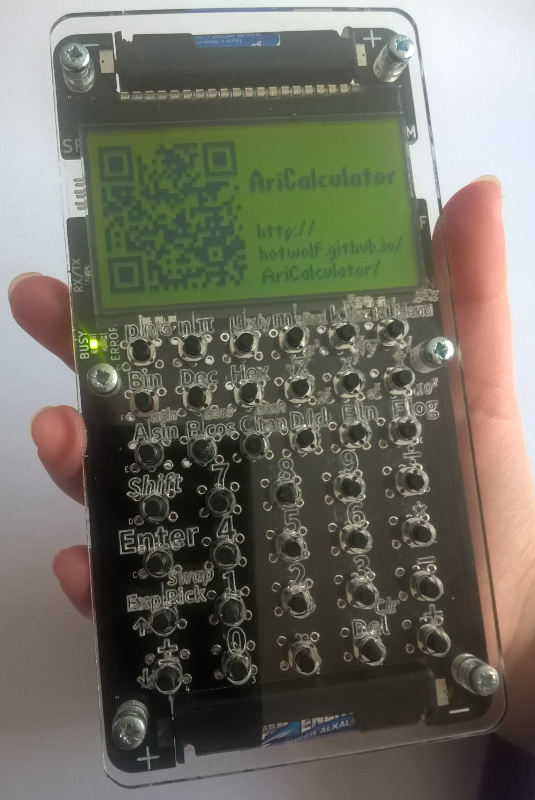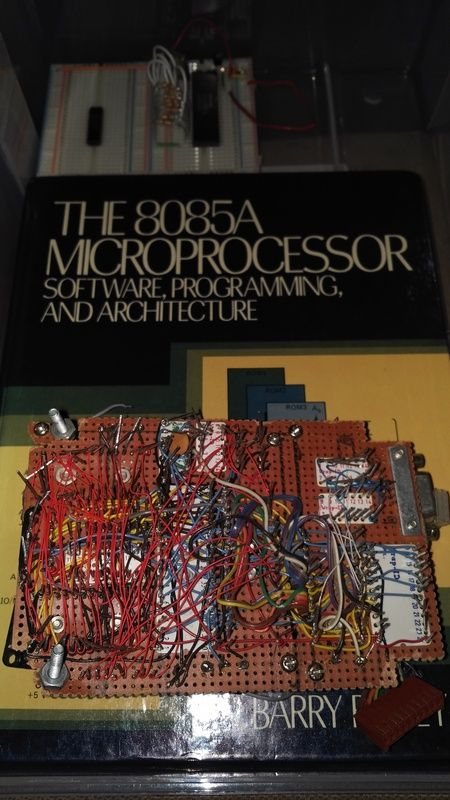# HP Forums

Full Version: AriCalculator is a home made pocket calculator.
You're currently viewing a stripped down version of our content. View the full version with proper formatting.
Pages: 1 2 3 4
This weekend I've built a black version of the AriCalculator:Now there is a little more contrast to the laser engravings.
(03-04-2017 06:29 PM)hotwolf Wrote: [ -> ]What does the back side look like?

It was old skool, wire wrappedThe breadboard at the top of the pic I'm starting over from scratch, its currently just wired to run NOP. I'm going to do a PCB in KiCAD and probably upload the final to OSHPark or something similar.

The DAC was so I could control the contrast like my 48sx, the serial port since my 48sx has a serial port. So it was influenced by my 48SX a good bit.
That wire wrapping is quite a piece of art.

Good luck with the rebuild, I hope all of your components are still intact.
Thats why I'm hunting down new parts. Bought a couple 8085's and 8155's and the 373 for the latch.
I like it in black too !
Can you sidelight that acrylic and make the key legends glow?
(03-07-2017 10:08 PM)EugeneNine Wrote: [ -> ]Can you sidelight that acrylic and make the key legends glow?

The calculator can be equipped with a back lit display. This circuit could be used to side light the front panel as well. The current PCB/panel hasn't been designed with provisions for these extra LEDs, but that could be hacked.

Front panel illumination is definitely something I will consider for the next revision of the calculator.
Amazing looking machine. Well done on a fantastic job Dirk.

Daniel
Daniel's four-function firmware is running on the real handheld device now:

http://hotwolf.github.io/AriCalculator/2...mware.html

I'm thinking of implementing e^x next. Maclaurin series converges rapidly for x near 0, but what about for other values of x?

One option is to factorise the index, e.g. e^32 = (e^3.2)^10, calculating e^3.2 using the Maclaurin series and then raising the result to the power of 10.

Another is to use e^x = e^a (1 + (x-a) +(1/2)(x-a)^2 + ...), storing e^5, e^10,...,e^30 in memory and choosing the closest value for a, e.g. e^32 = e^30 (1 + (32-30) + (1/2)(32-30)^2+...).

Thoughts?
(06-13-2017 03:20 AM)Dan Wrote: [ -> ]I'm thinking of implementing e^x next. Maclaurin series converges rapidly for x near 0, but what about for other values of x?

One option is to factorise the index, e.g. e^32 = (e^3.2)^10, calculating e^3.2 using the Maclaurin series and then raising the result to the power of 10.

Another is to use e^x = e^a (1 + (x-a) +(1/2)(x-a)^2 + ...), storing e^5, e^10,...,e^30 in memory and choosing the closest value for a, e.g. e^32 = e^30 (1 + (32-30) + (1/2)(32-30)^2+...).

Thoughts?

You can use a CORDIC algorithm to calculate the exponential function. This page gives C source code implementing the algorithm for a wide range of different functions. It should be faster than using the power series directly.

Nigel (UK)
The GNU bc utility uses $$e^{-x} = \frac{1}{e^x}$$ and $$e^x = { \left( e^{\frac{x}{2}} \right) }^2$$ to range reduce so that x ≤ 1.

Then it uses the Maclaurin series which will converge very quickly.

- Pauli
Very nice, thank you Nigel and Pauli.

This gives me quite a bit to work with. I like the idea of using e^x = (e^x/2)^2 to reduce the range to [-1,1] and then squaring the result repeatedly. I wonder why they use e^-x = 1/e^x to change the sign?
(06-15-2017 04:52 AM)Dan Wrote: [ -> ]I wonder why they use e^-x = 1/e^x to change the sign?

My initial thought would be due to accuracy issues, with x < 0, the series alternates in sign. I've seen the same thing done in other implementations of $$e^x$$.

You might want to consider using $$e^x = { \left( e^{\frac{x}{10}} \right) }^{10}$$ since you are working in base ten. It would also be possible to reduce the threshold below 1, this will increase the rate of convergence of the series expansion, i.e. reduce x until $$x < \frac{1}{10} = x_{max}$$ or even smaller. It becomes a balancing act between the costs of the preprocessing and the series computation. Accuracy loss can also come into play, so verify the algorithm on a desktop computer first.

You'll want to carry more digits than the result and ideally compute the series backwards using Horner's method. This means estimating the number of terms required in advance -- the worst case will be $$x = x_{max}$$ and since the first term in the expansion is 1, you'll need an n such that $$1 + \frac{{x_{max}}^n}{n!}$$ stays 1.

Pauli
(06-15-2017 04:52 AM)Dan Wrote: [ -> ]This version includes the four basic functions, factorial, square root (using Newton's/Babylonian method) some basic stack operations and the ability to view the contents of the stack memory (8 byte 10's complement BCD mantissa and 1 byte binary exponent).

Once you have the natural logarithm implemented, the gamma function could replace factorial.

Pauli
(06-15-2017 04:52 AM)Dan Wrote: [ -> ]Clive Maxfield wrote a great book on algorithms for the four basic functions ("How computers do math"), but it only deals with integers and I couldn't find anything that looks at floating-point numbers in similar detail.

There are quite a few things floating around the internet and in print. The last message on the first page of this thread lists some of the books I've found useful. The link to Kahan's site is invaluable for background. Also add in Knuth's Seminumerical Algorithms.

The WP 34S codebase implements a lot of decimal floating point functions. Other places to look are the source code for the GNU C library, the R statistics package, GNU's bc, the Cephes library -- these are all binary floating point based. The Intel decimal library is good but the algorithms aren't suitable for small processors (table based / using binary floating point functions as estimates).

CASIO's Keisan online calculator is good for checking results as is Wolfram's Alpha (by default it limits floating point accuracy which has caught me out).

- Pauli
I was able to significantly reduce execution time for the square root algorithm by using the "Friden" method instead of Newton's method. This algorithm was used in the Friden electro-mechanical calculator and is described in an HP journal from the 70's.

I will do some more timing and post the results.
That's a bit surprising.

What initial estimate did you use to start Newton's method? If this is moderately close, Newton's method converges very rapidly which generally compensates for the division involved.

Pauli
I thought the choice of CORDIC was more to do with it being tiny to implement and reusable between many transcendental functions. Polynomial and rational approximations tend to require a lot of constants. They also require repeated multiplications which could hurt performance, so it is believable that this was involved in the decision.

Newton's method for square root requires a division per iteration, but each iteration should double the number of correct digits. In other words, it converges very quickly if the initial estimate is close enough.

Pauli
Pages: 1 2 3 4
Reference URL's
• HP Forums: https://www.hpmuseum.org/forum/index.php
• :